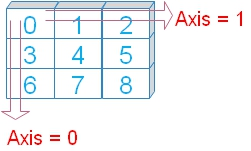# Pandas DataFrame std()

Pandas

``DataFrame.std(self, axis=None, skipna=None, level=None, ddof=1, numeric_only=None, **kwargs)``
We can get stdard deviation of DataFrame in rows or columns by using std().

 `self` array, elements to get the std value `axis` Int (optional ), or tuple, default is None, standard deviation among all the elements. If axis given then values across the axis is returned. `level` int ( Optional ),default is None, for multiindex Axis. count along the level. `skipna` Bool ( Optional ),default is True, Exclude NA values. `numeric_only` Bool ( Optional ),default is None, include only Int, floot and boolean columns. `ddof` Delta Degrees of Freedom ( default is 1 ) , N - ddof is used where N is the number of elements in computing the standard deviation
``````import pandas as pd
my_dict={
'id':[1,2,3,4,5,4,2],
'name':['John','Max','Arnold','Krish','John','Krish','Max'],
'class1':['Four','Three','Three','Four','Four','Four','Three'],
'mark':[75,85,55,60,60,60,85],
'sex':['female','male','male','female','female','female','male']
}
my_data = pd.DataFrame(data=my_dict)
print(my_data.std())``````
Output
``````id       1.414214
mark    12.817399
dtype: float64``````
Using only mark column ( with output )
``print(my_data['mark'].std()) # 12.817398889233116``

## Using axisWe will use option axis=0 ( default ) by adding to above code.

( The last line is only changed )
``print(my_data.std(axis=1))``
Along the horizontal row ( axis=1 ) the standard deviation among values of two columns ( id and Mark ) is calculated. For example for third row [3,55] is 36.769553.
Output is here.
``````0    52.325902
1    58.689863
2    36.769553
3    39.597980
4    38.890873
5    39.597980
6    58.689863
dtype: float64``````
``print(my_data.std(axis=0))``
Output
``````id       1.414214
mark    12.817399
dtype: float64``````

## ddof

`ddof = 0` this is Population Standard Deviation
`ddof = 1` ( default) , this is Sample Standard Deviation
``print(my_data.std(ddof=0))``
Output
``````id       1.309307
mark    11.866606
dtype: float64``````

## Handling NA data using skipna option

We will use skipna=True to ignore the null or NA data. Let us check what happens if it is set to True ( skipna=True )
``````import numpy as np
import pandas as pd
my_dict={'NAME':['Ravi','Raju','Alex','Ron','King','Jack'],
'ID':[1,2,3,4,5,6],
'MATH':[80,40,70,70,70,30],
'ENGLISH':[80,70,np.nan,50,60,30]}
my_data = pd.DataFrame(data=my_dict)
print(my_data.std(skipna=True))``````
Output
``````ID          1.870829
MATH       20.000000
ENGLISH    19.235384
dtype: float64``````

## numeric_only

Default value is None, we can set it to True ( numeric_only=True ) to include only float, int, boolean columns. We can included all by setting it to False ( numeric_only=False ) . Let us see the outputs .
``print(my_data.std(numeric_only=True))``
Output is same as above as we considered ID , MATH and ENGLISH columns. By changing to True we will get error message.
``print(my_data.std(numeric_only=False))``
TypeError: could not convert string to float: 'Ravi'

Subscribe to our YouTube Channel here

## Subscribe

* indicates required
Subscribe to plus2netplus2net.com